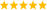Biomedical User Group Discussions

cancel
Showing results for
Did you mean:

Highlighted

How to calculate the pecentile of Amplitude probability distribution function for emg signal

Hi i want calculate the 10th, 50th and 90th percentile of Amplitude Probability distribution function (APDF) for an EMG data using labView... Can anyone help me, since am beginner in lab view

Message 1 of 3
(1,512 Views)

Re: How to calculate the pecentile of Amplitude probability distribution function for emg signal

@nawinerd wrote:

Can anyone help me, since am beginner in lab viewLabVIEW ChampionDo more with less code and in less timeMessage 2 of 3
(1,490 Views)

Re: How to calculate the pecentile of Amplitude probability distribution function for emg signal

Did you have any luck with this? I am about to attempt this also, but was wondering if there is any sample code around?

Message 3 of 3
(1,022 Views)